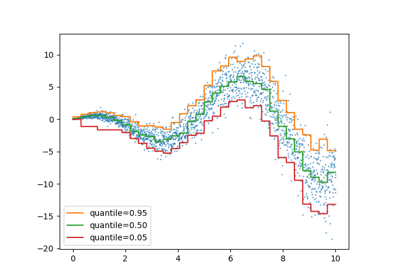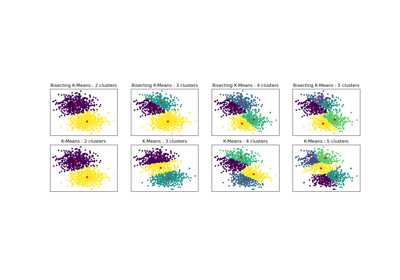# sklearn.cluster.BisectingKMeans¶

class sklearn.cluster.BisectingKMeans(n_clusters=8, *, init='random', n_init=1, random_state=None, max_iter=300, verbose=0, tol=0.0001, copy_x=True, algorithm='lloyd', bisecting_strategy='biggest_inertia')[source]

Bisecting K-Means clustering.

Read more in the User Guide.

New in version 1.1.

Parameters:
n_clustersint, default=8

The number of clusters to form as well as the number of centroids to generate.

init{‘k-means++’, ‘random’} or callable, default=’random’

Method for initialization:

‘k-means++’ : selects initial cluster centers for k-mean clustering in a smart way to speed up convergence. See section Notes in k_init for more details.

‘random’: choose n_clusters observations (rows) at random from data for the initial centroids.

If a callable is passed, it should take arguments X, n_clusters and a random state and return an initialization.

n_initint, default=1

Number of time the inner k-means algorithm will be run with different centroid seeds in each bisection. That will result producing for each bisection best output of n_init consecutive runs in terms of inertia.

random_stateint, RandomState instance or None, default=None

Determines random number generation for centroid initialization in inner K-Means. Use an int to make the randomness deterministic. See Glossary.

max_iterint, default=300

Maximum number of iterations of the inner k-means algorithm at each bisection.

verboseint, default=0

Verbosity mode.

tolfloat, default=1e-4

Relative tolerance with regards to Frobenius norm of the difference in the cluster centers of two consecutive iterations to declare convergence. Used in inner k-means algorithm at each bisection to pick best possible clusters.

copy_xbool, default=True

When pre-computing distances it is more numerically accurate to center the data first. If copy_x is True (default), then the original data is not modified. If False, the original data is modified, and put back before the function returns, but small numerical differences may be introduced by subtracting and then adding the data mean. Note that if the original data is not C-contiguous, a copy will be made even if copy_x is False. If the original data is sparse, but not in CSR format, a copy will be made even if copy_x is False.

algorithm{“lloyd”, “elkan”}, default=”lloyd”

Inner K-means algorithm used in bisection. The classical EM-style algorithm is "lloyd". The "elkan" variation can be more efficient on some datasets with well-defined clusters, by using the triangle inequality. However it’s more memory intensive due to the allocation of an extra array of shape (n_samples, n_clusters).

bisecting_strategy{“biggest_inertia”, “largest_cluster”}, default=”biggest_inertia”

Defines how bisection should be performed:

• “biggest_inertia” means that BisectingKMeans will always check

all calculated cluster for cluster with biggest SSE (Sum of squared errors) and bisect it. This approach concentrates on precision, but may be costly in terms of execution time (especially for larger amount of data points).

• “largest_cluster” - BisectingKMeans will always split cluster with

largest amount of points assigned to it from all clusters previously calculated. That should work faster than picking by SSE (‘biggest_inertia’) and may produce similar results in most cases.

Attributes:
cluster_centers_ndarray of shape (n_clusters, n_features)

Coordinates of cluster centers. If the algorithm stops before fully converging (see tol and max_iter), these will not be consistent with labels_.

labels_ndarray of shape (n_samples,)

Labels of each point.

inertia_float

Sum of squared distances of samples to their closest cluster center, weighted by the sample weights if provided.

n_features_in_int

Number of features seen during fit.

feature_names_in_ndarray of shape (n_features_in_,)

Names of features seen during fit. Defined only when X has feature names that are all strings.

KMeans

Original implementation of K-Means algorithm.

Notes

It might be inefficient when n_cluster is less than 3, due to unnecessary calculations for that case.

Examples

>>> from sklearn.cluster import BisectingKMeans
>>> import numpy as np
>>> X = np.array([[1, 2], [1, 4], [1, 0],
...               [10, 2], [10, 4], [10, 0],
...               [10, 6], [10, 8], [10, 10]])
>>> bisect_means = BisectingKMeans(n_clusters=3, random_state=0).fit(X)
>>> bisect_means.labels_
array([2, 2, 2, 0, 0, 0, 1, 1, 1], dtype=int32)
>>> bisect_means.predict([[0, 0], [12, 3]])
array([2, 0], dtype=int32)
>>> bisect_means.cluster_centers_
array([[10.,  2.],
[10.,  8.],
[ 1., 2.]])


Methods

 fit(X[, y, sample_weight]) Compute bisecting k-means clustering. fit_predict(X[, y, sample_weight]) Compute cluster centers and predict cluster index for each sample. fit_transform(X[, y, sample_weight]) Compute clustering and transform X to cluster-distance space. get_feature_names_out([input_features]) Get output feature names for transformation. get_params([deep]) Get parameters for this estimator. Predict which cluster each sample in X belongs to. score(X[, y, sample_weight]) Opposite of the value of X on the K-means objective. set_params(**params) Set the parameters of this estimator. Transform X to a cluster-distance space.
fit(X, y=None, sample_weight=None)[source]

Compute bisecting k-means clustering.

Parameters:
X{array-like, sparse matrix} of shape (n_samples, n_features)

Training instances to cluster.

Note

The data will be converted to C ordering, which will cause a memory copy if the given data is not C-contiguous.

yIgnored

Not used, present here for API consistency by convention.

sample_weightarray-like of shape (n_samples,), default=None

The weights for each observation in X. If None, all observations are assigned equal weight.

Returns:
self

Fitted estimator.

fit_predict(X, y=None, sample_weight=None)[source]

Compute cluster centers and predict cluster index for each sample.

Convenience method; equivalent to calling fit(X) followed by predict(X).

Parameters:
X{array-like, sparse matrix} of shape (n_samples, n_features)

New data to transform.

yIgnored

Not used, present here for API consistency by convention.

sample_weightarray-like of shape (n_samples,), default=None

The weights for each observation in X. If None, all observations are assigned equal weight.

Returns:
labelsndarray of shape (n_samples,)

Index of the cluster each sample belongs to.

fit_transform(X, y=None, sample_weight=None)[source]

Compute clustering and transform X to cluster-distance space.

Equivalent to fit(X).transform(X), but more efficiently implemented.

Parameters:
X{array-like, sparse matrix} of shape (n_samples, n_features)

New data to transform.

yIgnored

Not used, present here for API consistency by convention.

sample_weightarray-like of shape (n_samples,), default=None

The weights for each observation in X. If None, all observations are assigned equal weight.

Returns:
X_newndarray of shape (n_samples, n_clusters)

X transformed in the new space.

get_feature_names_out(input_features=None)[source]

Get output feature names for transformation.

Parameters:
input_featuresarray-like of str or None, default=None

Only used to validate feature names with the names seen in fit.

Returns:
feature_names_outndarray of str objects

Transformed feature names.

get_params(deep=True)[source]

Get parameters for this estimator.

Parameters:
deepbool, default=True

If True, will return the parameters for this estimator and contained subobjects that are estimators.

Returns:
paramsdict

Parameter names mapped to their values.

predict(X)[source]

Predict which cluster each sample in X belongs to.

Prediction is made by going down the hierarchical tree in searching of closest leaf cluster.

In the vector quantization literature, cluster_centers_ is called the code book and each value returned by predict is the index of the closest code in the code book.

Parameters:
X{array-like, sparse matrix} of shape (n_samples, n_features)

New data to predict.

Returns:
labelsndarray of shape (n_samples,)

Index of the cluster each sample belongs to.

score(X, y=None, sample_weight=None)[source]

Opposite of the value of X on the K-means objective.

Parameters:
X{array-like, sparse matrix} of shape (n_samples, n_features)

New data.

yIgnored

Not used, present here for API consistency by convention.

sample_weightarray-like of shape (n_samples,), default=None

The weights for each observation in X. If None, all observations are assigned equal weight.

Returns:
scorefloat

Opposite of the value of X on the K-means objective.

set_params(**params)[source]

Set the parameters of this estimator.

The method works on simple estimators as well as on nested objects (such as Pipeline). The latter have parameters of the form <component>__<parameter> so that it’s possible to update each component of a nested object.

Parameters:
**paramsdict

Estimator parameters.

Returns:
selfestimator instance

Estimator instance.

transform(X)[source]

Transform X to a cluster-distance space.

In the new space, each dimension is the distance to the cluster centers. Note that even if X is sparse, the array returned by transform will typically be dense.

Parameters:
X{array-like, sparse matrix} of shape (n_samples, n_features)

New data to transform.

Returns:
X_newndarray of shape (n_samples, n_clusters)

X transformed in the new space.

## Examples using sklearn.cluster.BisectingKMeans¶Release Highlights for scikit-learn 1.1

Release Highlights for scikit-learn 1.1Bisecting K-Means and Regular K-Means Performance Comparison

Bisecting K-Means and Regular K-Means Performance Comparison